# Media Control Using Hand Gestures

A system which operates some commands when a specific hand gesture is being detected.

IntermediateFull instructions provided5 hours321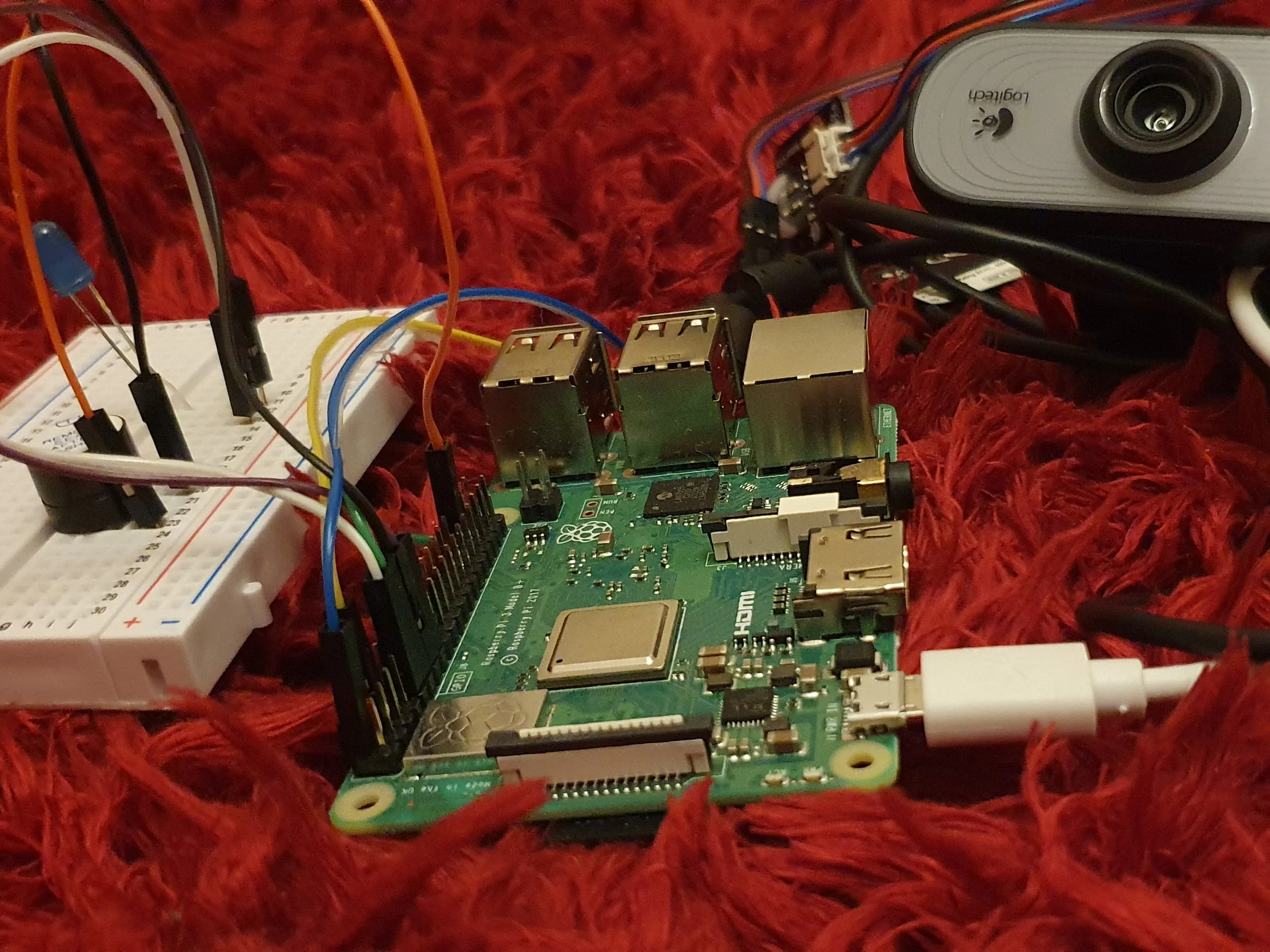## Things used in this project

### Hardware componentsRaspberry Pi 3 Model B
×1LED (generic)
×1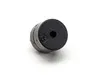Buzzer
×1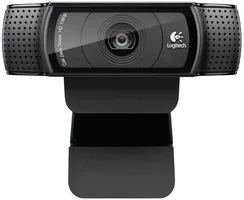Webcam, Logitech® HD Pro
×1×1Jumper wires (generic)
×1

### Software apps and online services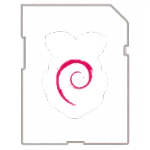Raspberry Pi Raspbian

## Schematics

### Complete Circuit

This board shows the connected wires of LED, and Buzzer and webcam all connected to RaspberryPi.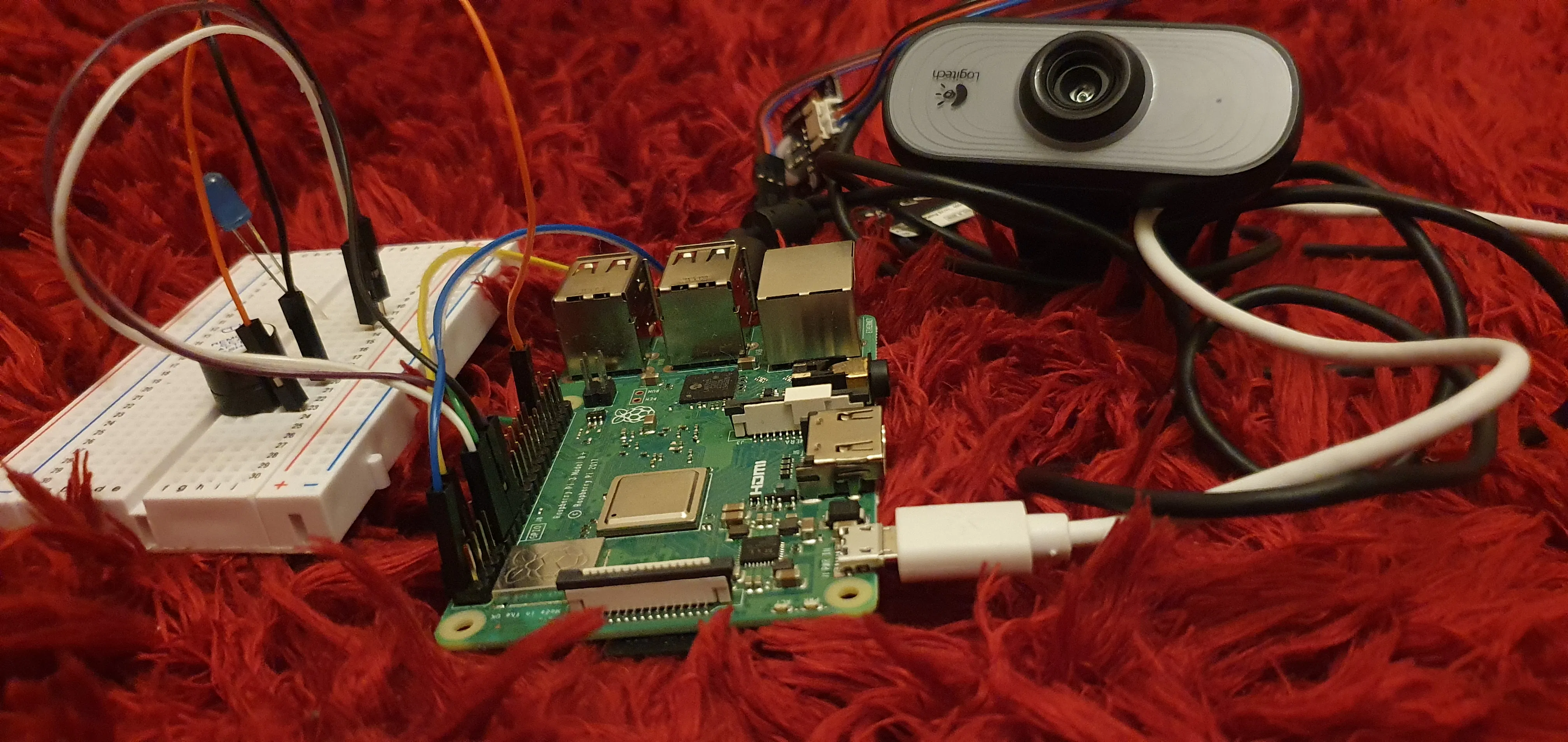Connection of Buzzer and LED to breadboard.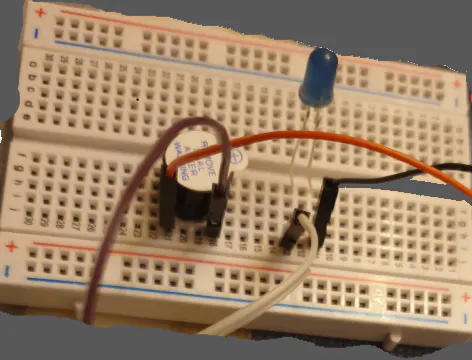### Circuit Board

The board without webcam attachment for clear view.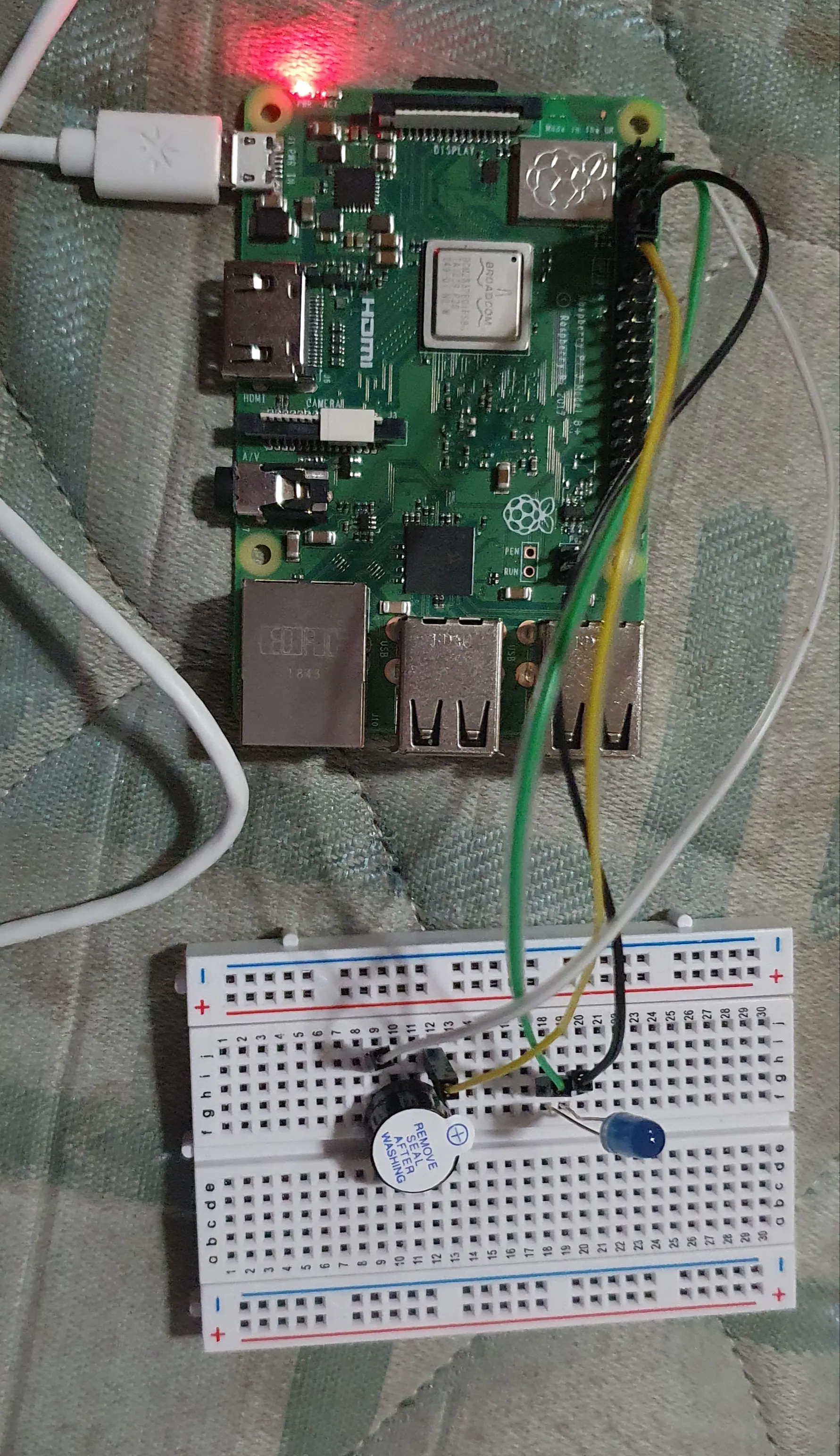## Code

### Prediction.py

Python
The main file used for real time prediction. Make sure to edit it to change the video path and the path of the saved model to the path in your computer.
```import vlc
import cv2
from tensorflow import keras
import tensorflow as tf
import operator
import numpy
import time
import RPi.GPIO as GPIO

GPIO.setwarnings(False)
GPIO.setmode(GPIO.BOARD)
GPIO.setup(11,GPIO.OUT)
GPIO.setup(13, GPIO.OUT)
from time import sleep

Instance = vlc.Instance()
player = Instance.media_player_new()
player.set_media(Media)
player.play()

cap = cv2.VideoCapture(0)
# Category dictionary
class_labels = ["NONE","ONE",'TWO','THREE','FOUR','FIVE']
while True:
# Simulating mirror image
frame = cv2.flip(frame, 1)

#getting roi of the hand part
x1 = 400
y1 = 50
x2 = 600
y2 = 300
cv2.rectangle(frame , (x1-2,y1-2),(x2+2,y2+2),(0,255,0),2)

# extracting the roi and converting it to gray
roi = frame[y1:y2 , x1:x2]
roi = cv2.resize(roi,(150,150))
roi = cv2.cvtColor(roi,cv2.COLOR_BGR2GRAY)

# applying a threshold to the region of interest
ret,test_image = cv2.threshold(roi,127,255,cv2.THRESH_BINARY_INV)
cv2.imshow('test',test_image)
result = classifier.predict(test_image.reshape(1, 150, 150, 1))
prediction = {'ONE': result,
'TWO': result,
'THREE': result,
'FOUR': result,
'FIVE': result}
# Sorting based on top prediction
prediction = sorted(prediction.items(), key=operator.itemgetter(1), reverse=True)

# Displaying the predictions
cv2.putText(frame, prediction, (100, 450), cv2.FONT_HERSHEY_PLAIN, 4, (0,0,255), 4)
print(prediction, )
if prediction == "THREE":
player.play()
GPIO.output(11,True)
GPIO.output(13,False)
elif prediction == "FOUR":
player.stop()
GPIO.output(11,False)
GPIO.output(13,True)

cv2.imshow("Frame", frame)

interrupt = cv2.waitKey(1)
if interrupt & 0xFF == 27: # esc key
break
cap.release()
cv2.destroyAllWindows()
```

### collect_data.py

Python
Generally used to take new images as our data. If you want to make your own custom data, then run this script else no need.
```import numpy as np
import tensorflow as tf
import cv2
from tensorflow import keras
import os
import operator

'''Data Collection Process'''
def collect_data():
# creating a directory for the captured images
if not os.path.exists("Images"):
os.makedirs("Images/train")
os.makedirs("Images/test")
os.makedirs("Images/train/None")
os.makedirs("Images/train/1")
os.makedirs("Images/train/2")
os.makedirs("Images/train/3")
os.makedirs("Images/train/4")
os.makedirs("Images/train/5")
os.makedirs("Images/test/None")
os.makedirs("Images/test/1")
os.makedirs("Images/test/2")
os.makedirs("Images/test/3")
os.makedirs("Images/test/4")
os.makedirs("Images/test/5")

mode = 'Train'
directory = "Images/Images/"+mode+"/"

cap = cv2.VideoCapture(0) #capturing the video
while (True):
frame = cv2.flip(frame,1)

# making dict ti get number of images of each categories
count = {"None" : len(os.listdir(directory+"None")),
"One" : len(os.listdir(directory+"/1")),
"Two" : len(os.listdir(directory+"/2")),
"Three" : len(os.listdir(directory+"/3")),
"Four" : len(os.listdir(directory+"/4")),
"Five" : len(os.listdir(directory+"/5"))
}

height = str(cap.get(cv2.CAP_PROP_FRAME_HEIGHT)) # 480
width = str(cap.get(cv2.CAP_PROP_FRAME_WIDTH))  # 640

# writing the counts of specified no of trained images on video
if ret == True:
cv2.putText(frame,mode,(10,40),cv2.FONT_HERSHEY_COMPLEX,1,(0,0,255),1,cv2.LINE_AA)
cv2.putText(frame,"One: "+str(count['One']),(10,100),cv2.FONT_HERSHEY_COMPLEX,1,(255,0,0),1,cv2.LINE_AA)
cv2.putText(frame,"Two:"+str(count['Two']),(10,140),cv2.FONT_HERSHEY_COMPLEX,1,(255,0,0),1,cv2.LINE_AA)
cv2.putText(frame,"Three:"+str(count['Three']),(10,180),cv2.FONT_HERSHEY_COMPLEX,1,(255,0,0),1,cv2.LINE_AA)
cv2.putText(frame,"Four:"+str(count['Four']),(10,220),cv2.FONT_HERSHEY_COMPLEX,1,(255,0,0),1,cv2.LINE_AA)
cv2.putText(frame,"Five:"+str(count['Five']),(10,260),cv2.FONT_HERSHEY_COMPLEX,1,(255,0,0),1,cv2.LINE_AA)

#creating a roi
x1 = 400
y1 = 50
x2 = 600
y2 = 300
cv2.rectangle(frame , (x1-2,y1-2),(x2+2,y2+2),(0,255,0),2)

# extracting the roi and converting it to gray
roi = frame[y1:y2 , x1:x2]
roi = cv2.resize(roi,(150,150))
roi = cv2.cvtColor(roi,cv2.COLOR_BGR2GRAY)
# applying a threshold to the region of interest
ret,roi = cv2.threshold(roi,127,255,cv2.THRESH_BINARY_INV)

#performing image processing dilation , filtering and smoothing
kernal = np.ones((2,2),np.uint8)
roi = cv2.dilate(roi,kernel=kernal ,iterations=1)
roi = cv2.erode(roi ,kernel=kernal ,iterations=1)
#roi = cv2.bilateralFilter(roi,9,75,75)
roi = cv2.medianBlur(roi,5)

cv2.imshow('ROI',roi)
cv2.imshow("frame",frame)

# commands dependent on keys pressed
k = cv2.waitKey(1)
if k == 27:
print("Escape closing camera")
break
elif k == ord('0'):
cv2.imwrite(directory+"None/"+str(count['None'])+".png",roi)
print("Picture labelled None saved to train!")
elif k == ord('1'):
cv2.imwrite(directory+"1/"+str(count['One'])+".png",roi)
print("Picture labelled 1 saved to train!")
elif k == ord('2'):
cv2.imwrite(directory+"2/"+str(count['Two'])+".png",roi)
print("Picture labelled 2 saved to train!")
elif k == ord('3'):
cv2.imwrite(directory+"3/"+str(count['Three'])+".png",roi)
print("Picture labelled 3 saved to train!")
elif k == ord('4'):
cv2.imwrite(directory+"4/"+str(count['Four'])+".png",roi)
print("Picture labelled 4 saved to train!")
elif k == ord('5'):
cv2.imwrite(directory+"5/"+str(count['Five'])+".png",roi)
print("Picture labelled 5 saved to train!")
else:
break
cap.release()
cv2.destroyAllWindows()
```

### train.py

Python
Creates a model, trains it and then saves the trained model to your system.
```def train_data():
# creating the CNN model
model = tf.keras.Sequential([
tf.keras.layers.Conv2D(32,(3,3),activation='relu',input_shape=(150,150,1)),
tf.keras.layers.MaxPool2D(2,2),
tf.keras.layers.Conv2D(32,(3,3),activation='relu'),
tf.keras.layers.MaxPool2D(2,2),
tf.keras.layers.Conv2D(32,(3,3),activation='relu'),
tf.keras.layers.MaxPool2D(2,2),
tf.keras.layers.Flatten(),
tf.keras.layers.Dense(512,activation='relu'),
tf.keras.layers.Dense(6,activation='softmax')
])

model.summary()

# compiling the model

# preparing the data and training the model
from tensorflow.keras.preprocessing.image import ImageDataGenerator
train_datagen = ImageDataGenerator(rescale=1./255)
test_datagen = ImageDataGenerator(rescale=1./255)

# stream train images from train directory
training_set = train_datagen.flow_from_directory(
'Images/Images/train',
target_size=(150,150),
batch_size=5,
color_mode = 'grayscale',
class_mode = 'categorical')

# stream test images from test directory
test_set = test_datagen.flow_from_directory(
'Images/Images/test',
target_size=(150,150),
batch_size=5,
color_mode = 'grayscale',
class_mode = 'categorical')

# fitting the training data to the model
model.fit_generator(
training_set,
steps_per_epoch = 40,
epochs=5,
validation_data=test_set,
#validation_steps=40,
verbose =2
)

#saving the model
model.save('model.h5')
```

## Credits

### Sibtain Reza

1 project • 0 followers